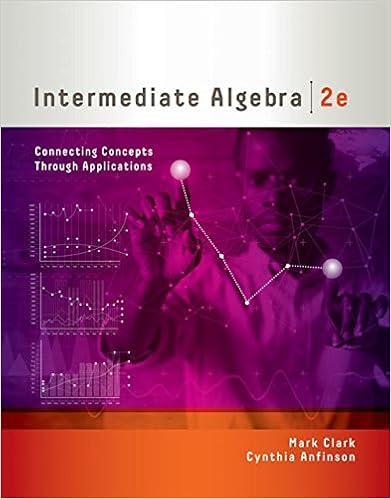# Refer to figure 3 4 if the price is 10 a there would

• Homework Help
• 9
• 94% (90) 85 out of 90 people found this document helpful

This preview shows page 4 - 7 out of 9 pages.

##### We have textbook solutions for you!
The document you are viewing contains questions related to this textbook.The document you are viewing contains questions related to this textbook.
Chapter 1 / Exercise 29
Intermediate Algebra: Connecting Concepts through Applications
ClarkExpert Verified
18) Refer to Figure 3-4.If the price is \$10,A) there would be a surplus of 600 units.B) there would be a shortage of 600 units.C) there would be a surplus of 200 units.D) there would be a shortage of 200 units.
19) Refer to Figure 3-4. At a price of \$10, how many units will be sold?
20) Refer to Figure 3-4. If the current market price is \$10, the market will achieve equilibrium by
##### We have textbook solutions for you!
The document you are viewing contains questions related to this textbook.The document you are viewing contains questions related to this textbook.
Chapter 1 / Exercise 29
Intermediate Algebra: Connecting Concepts through Applications
ClarkExpert Verified
Figure 3-621) Refer to Figure 3-6. The figure above represents the market for canvas tote bags. Assume that the market price is \$35. Which of the following statement is true?
22) Which of the following would cause both the equilibrium price and equilibrium quantity of cotton (assume that cotton is a normal good) to increase? A) an increase in consumer incomeB) a drought that sharply reduces cotton outputC) a decrease in consumer incomeD) unusually good weather that results in a bumper crop of cotton
23) Which of the following would cause the equilibrium price of white bread to decrease and the equilibrium quantity of white bread to increase?
24) Assume that both the demand curve and the supply curve for MP3 players shift to the right but the demand curve shifts more than the supply curve. As a result
•••